data visualization with Python Archives - DexLab Analytics | Big Data Hadoop SAS R Analytics Predictive Modeling & Excel VBA

## Visualization with Python Part-V: Introducing the Pandas_bokeh libraryIn our fifth installment of the visualization series using Python programming language, we introduce you to another powerful library in Python that is the Pandas_bokeh library. So, let’s find out what you can achieve with Pandas_bokeh library.

Pandas_bokeh is a library which can help you create interactive graphs in python. One can zoom in, zoom out, select a certain portion of the graph to see, move the plot left, right and center, create tabs in case they want to see a single plot at a time, create multiple plots at a time, create widgets like dropdown list, check boxes, radio buttons, slider etc. It is similar to the shiny app which is used in the r programming language  but simpler and faster.

#### How to install pandas_bokeh?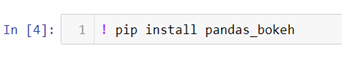In the above code we are changing our jupyter notebook code cell into a command line by using ! and then we can use pip (python installation package) to install the library.

#### How to create a simple line plot using pandas_bokeh ?

The first thing to do is import the libraries which we will be using to create a line plot.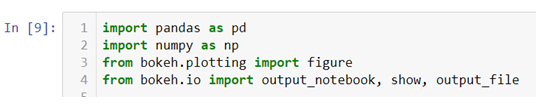In the above line of codes we are creating two separate data frames df_d and df_d1 each containing Monday and Friday’s sales and dates separately now all we need to do is build a canvas using .figure() method and use few other arguments like x_axis_type to define the data type of the x axis and x_axis_label and y_axis_label to set graph labels, to adjust width and height of the canvas we have used plot_width and plot_height argument and to set title and title location we have used title and title_location. Once we have our canvas ready we can use .line() method and add x axis and y axis data to plot our graphs.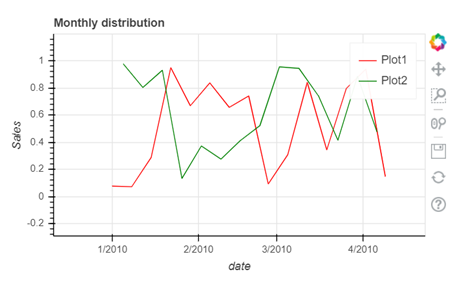• To interact with your graph you can use the icons on the right hand side corner which will help you zoom in and out, look at a certain part of the graph, scroll to zoom in and out, save your plot and reset the changes made by you using the side icons.

At the end of this segment you must have become familiar with the nuances of the Pandas_bokeh library. As you continue on with the series, you will realize that you are becoming an expert in visualization. On Dexlab Analytics blog, you will find interesting blogs on various topics related to Python certification training.

.

## Visualization with Python Part IV: Learn To Create A Box Plot Using Seaborn Library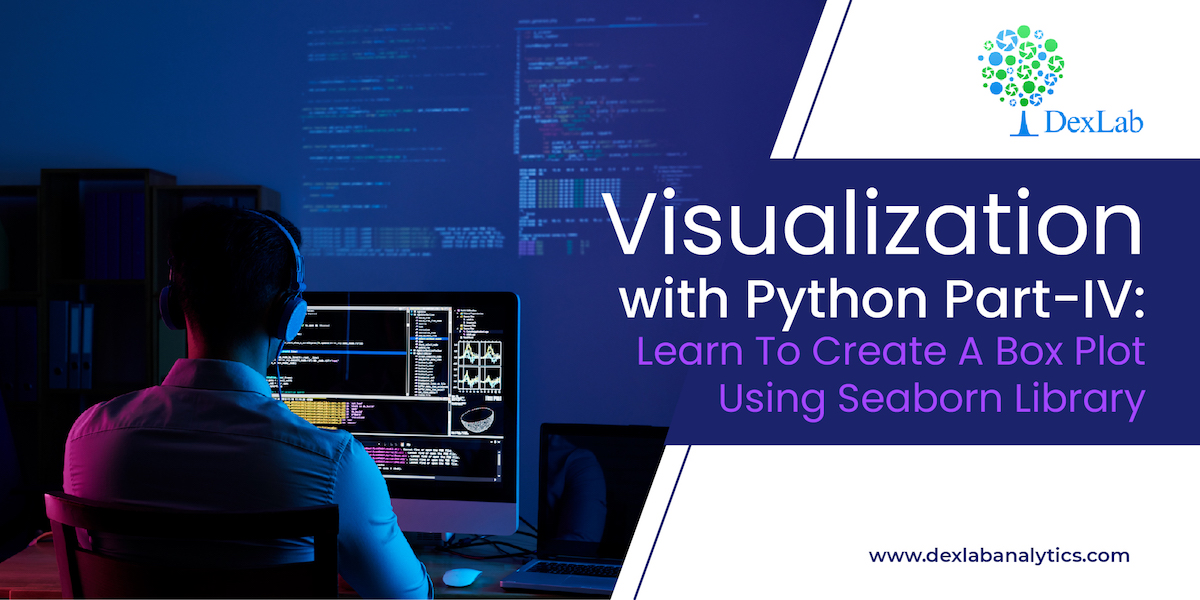This is the 4th part of the series on visualization using Python programming language, where we will continue our discussion on the Seaborn library. Now that you have become familiar with the basics of the Seaborn library, you will be learning specific skills such as learning to create a box plot using Seaborn. So, let’s begin.

Seaborn library offers a list of pre-defined methods to create semi-flexible plots in Python and one of them is. boxplot() method. But what is a box plot?

Answer:- A box plot often known as box and whisker plot is a graph created to visualize the distribution of numerical data and skewness through displaying the data quartiles (or percentiles) and median.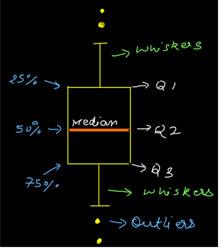Creating a box plot

Let’s begin by importing the Seaborn and Matplotlib library.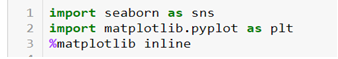We will again be using the tips dataset which is a pre-defined dataset in Seaborn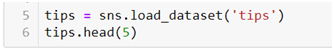Data description:- This is a dataset of a restaurant which keeps a record of the amount of bill paid by a customer, tip amount over the total bill paid, gender of the customer, whether he or she was a smoker or not, the day on which they ate at the restaurant, what was the time when they ate at the restaurant and the size of the table they booked.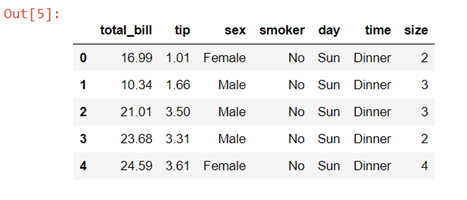To create a box plot we will be using .boxplot() method.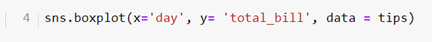On the x axis we have day column having categorical data type and on the y axis we have total_bill column having numerical data type. Thus for each day with the help of a box plot we will  be able to visualize how the total_bill changes around its median value.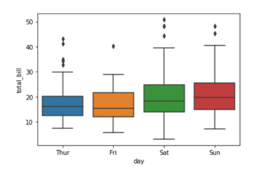To add title to the graph we can use. title() method from the Matplotlib library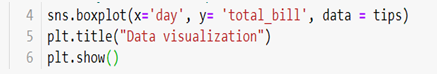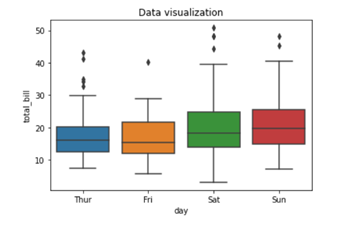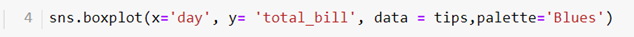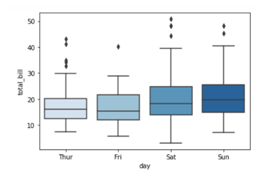We are adding a list of palette colors in this blog down below:-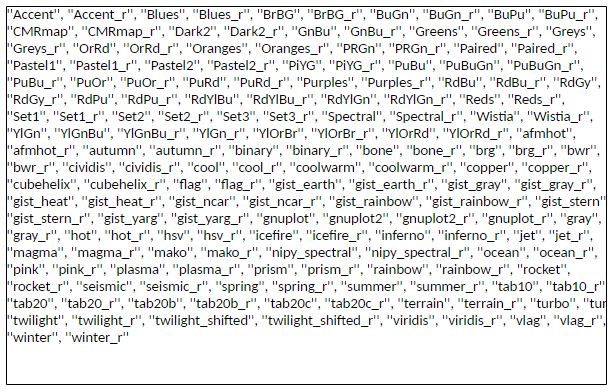You can replace the color mentioned in the above code to see which color variations you would prefer in your graph. For example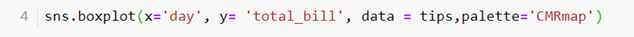Here we are using color palette ‘CMRmap’ to change my graph color from different shades of blues to a completely different color range i.e. from blues to orange, violet, pale yellow etc.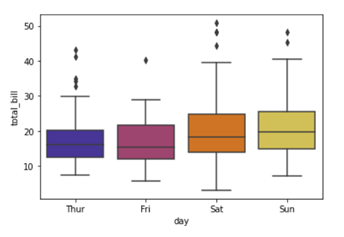This tutorial hopefully, has clarified the concept and you can now create boxplots with Seaborn. Since this is a series you need to keep track of all the parts to be a visualization expert as we take you through the process step by step. Follow the Dexlab Analytics blog to access more informative posts on different topics including python for data analysis.

Go through the video tutorial attached below to get more in-depth knowledge.

.

## Visualization with Python Part III: Introducing The Seaborn Library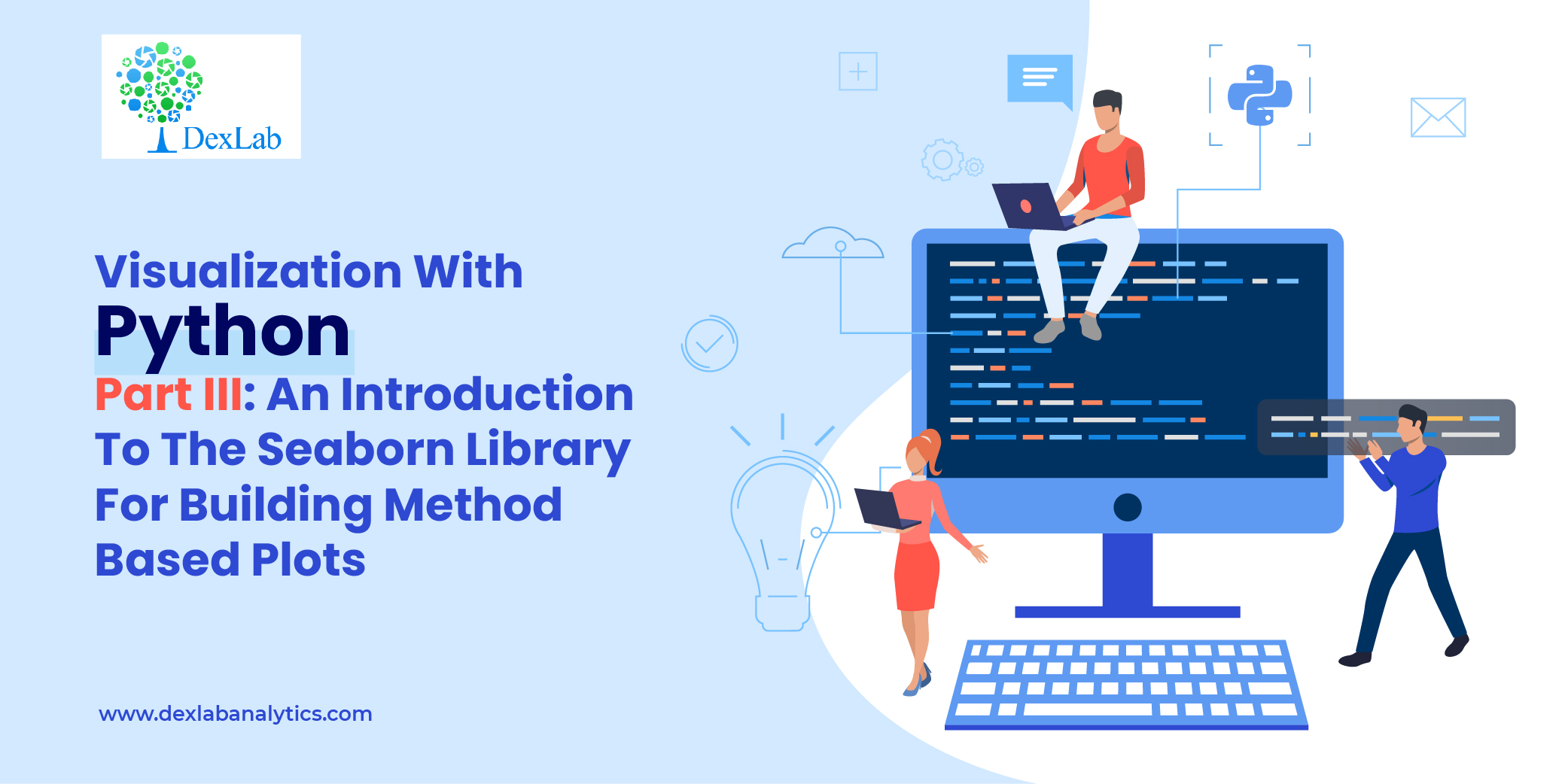In this 3rd part of the visualization series using Python programming language, we are going to introduce you to the Seaborn Library. Seaborn is a visualization library which is built on top of Matplotlib library in Python. This library helps us build method based plots which when combined with Matplotlib library methods lets us build flexible graphs.

In this tutorial we will be using tips data, which is a pre-defined dataset in the Seaborn library.

So let’s begin by importing the Seaborn library and giving it a sudo name sns. We will also be importing Matplotlib library to add more attributes to our graphs.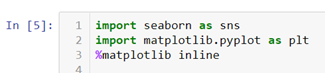To load the tips data set we will be using .load_dataset() method.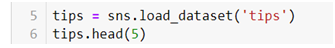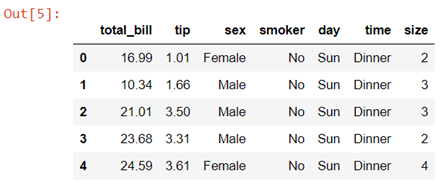Data description:- This is a dataset of a restaurant which keeps a record of the amount of bill paid by a customer, tip amount over the total bill paid, gender of the customer, whether he or she was a smoker or not, the day on which they ate at the restaurant, what was the time when they ate at the restaurant and the size of the table they booked.

In case while loading the dataset you see a warning box appear on the screen you don’t need to worry , you haven’t done anything wrong. These FutureWarning boxes appear to make you aware that in the future there might be some changes in the library or methods you are using.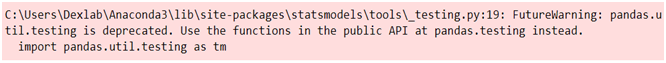You can simply use the .simplefilter() method from the warning library to make them disappear.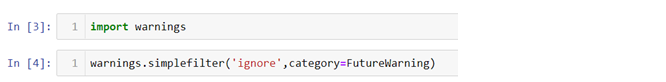Here the category argument helps you decide which type of warning you want to ignore.

#### Creating a bar plot in Seaborn

Now let’s quickly go ahead and create a bar plot with the help of .barplot() method.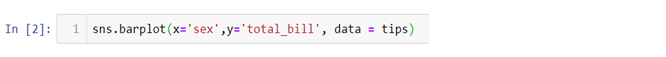In the above line of code we are using column named sex on the x axis and total_bill on the y axis. But this bar plot is very different from the bar plot which we usually make. The basic concept of a bar plot is to check the frequency but here we are also mentioning the y axis data which in general is not the case with a normal bar plot, that is, we get the frequency on the y axis when we are plotting a bar graph. So what does the above code do?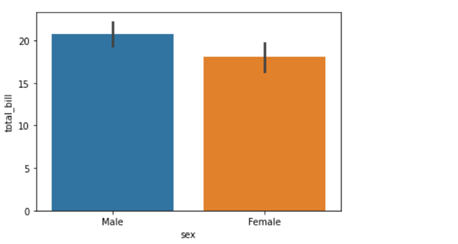The above code compares the average of the category. In our case the above graph shows that the average bill of male is higher than the average bill of female. In case you want to plot a graph showing the average variation of bill around the mean (Standard deviation) you can use estimator argument within the .barplot() to do so.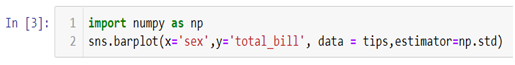Also if you want to change the background of your graph you can easily do so by using .set_style() method.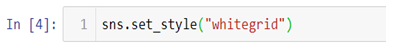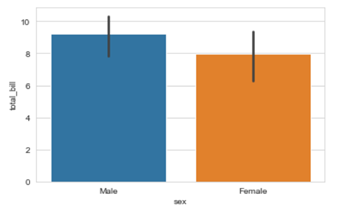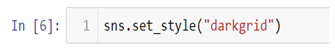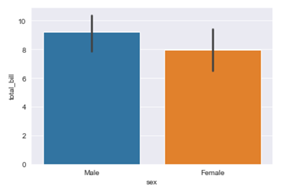The vertical bars between the graph are called the error bars and they tell you how far from your mean or standard deviation by max data varies.

Matplotlib methods can be imported and added to the Seaborn graphs to make them more presentable and flexible. Here we will be adding a title in our graph by using .title() method from the Matplotlib library.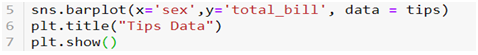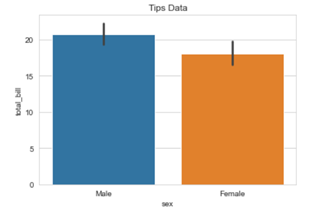You can use other Matplotlib methods like .legend(),xlabel(),.ylabel() etc,. to add more value to your graphs.

The video tutorial attached below will further help you clarify your ideas regarding the Seaborn library. Follow the series to gain expertise in visualization with Python programming language. Keep on following the Dexlab Analytics blog for reading more informative posts on Python for data science training.

.

## An Introduction to Matplotlib Object Oriented Method: Visualization with Python (Part II)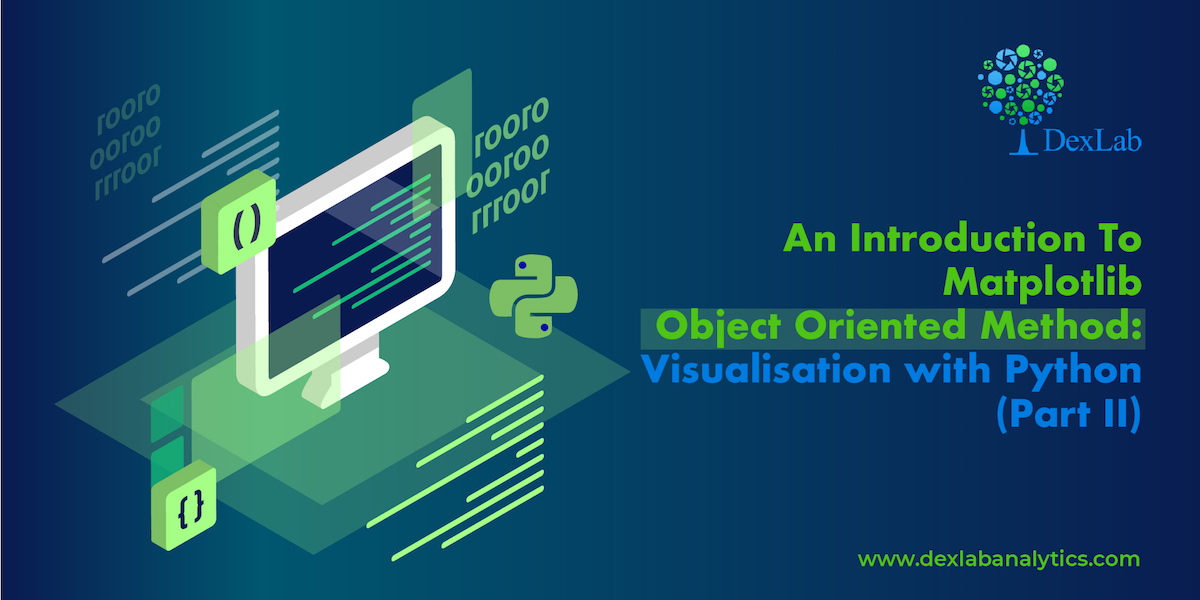In the last blog that covered Part 1 of the visualization series using Python programming language, we have learned the basics of the Matplotlib Library. Now that our grasp on the basics is strong we would move further. Let’s break it all down with a more formal introduction of Matplotlib’s Object Oriented API. This means we will instantiate figure objects and then call methods or attributes from that object.

### Introduction to the Object Oriented Method

The main idea in using the more formal Object Oriented method is to create figure objects and then just call methods or attributes off of that object. This approach is nicer when dealing with a canvas that has multiple plots on it.

#### How to make multiple plots using .add_axes()

To begin, we create a figure instance. Then we can add axes to that figure where .figure()is a method which helps us create an empty canvas and then we use .add_axes() method to give the position where the plot is to be made. The positional arguments [left, bottom, width, height] help us decide from where the graph should begin within the canvas and what should be the width and the height of the graph. Since the area of the graph is 100% (1.0), the range of the positional argument should be between 0 and 1 and in case you want to plot the graph half within and half outside the canvas, you can go beyond the specified range depending upon your needs.

Let’s  quickly get to the coding part now.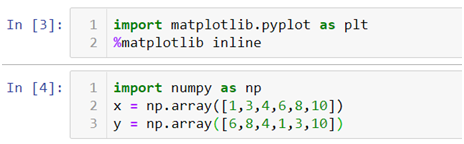• In the above line of codes we are simply importing the Matplotlib library and creating a data which we want to plot.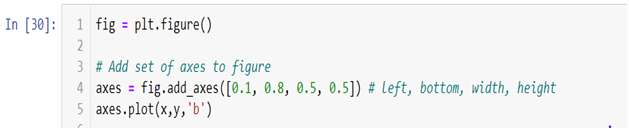• plt.figure()method is helping us create an empty canvas and then we are giving the positional values to the .add_axes() method. As you can see we are using a variable named fig to save our canvas and then using the same variable as an object to add an axes to the canvas. Now all we need to do is use that axes to build are graph by adding x and y data.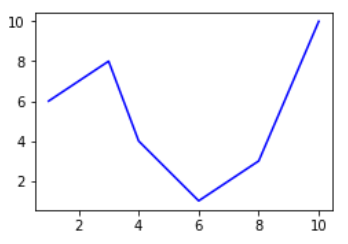• Now you must be wondering why we aren’t able to see a plot in the corner of the canvas? It is because this procedure works only if we were to build multiple plots. So now let’s see how we can use the .add_axes() method to build multiple plots on top of each other.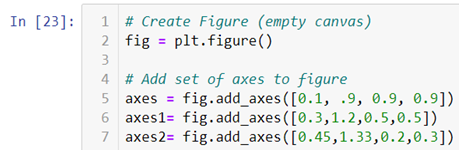• In the above line of codes we are creating three axes and each axes is smaller than the other so that we are able to plot multiple graphs on top of each other.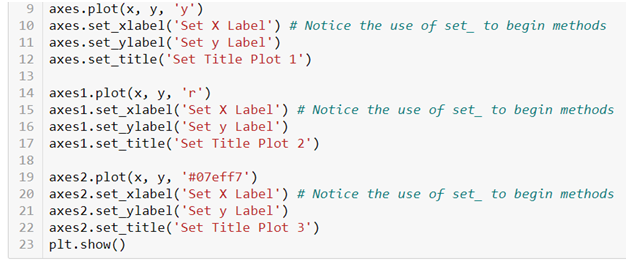• Here we are making three different graphs and each graph has its own title and x axis and y axis labels. But to add title and axis labels we are now using .set_title(), .set_xlabel(), and .set_ylabel() instead of .title(), .xlabel() and .ylabel(). In axes2.plot() we are also using RGB color instead of using the predefined color in .plot() method. You can use your favorite color too by simply typing RGB color picker in your Google search and copy pasting the color code in the .plot() method. After running the above code we get the following graph:-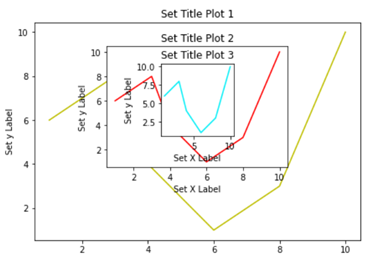#### How to make multiple plots using .subplots()

.subplots() method is similar to the previous .subplot() method, the only difference is that now we use it on a canvas.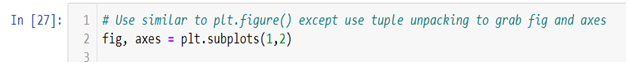Do not forget to check out the video tutorial attached below to learn how this method works. Keep following the series to upgrade your skills and to explore more informative posts on topics like Python Programming training you need to follow the Dexlab Analytics blog.

.

## A Quick Guide To Using Matplotlib Library (Part I)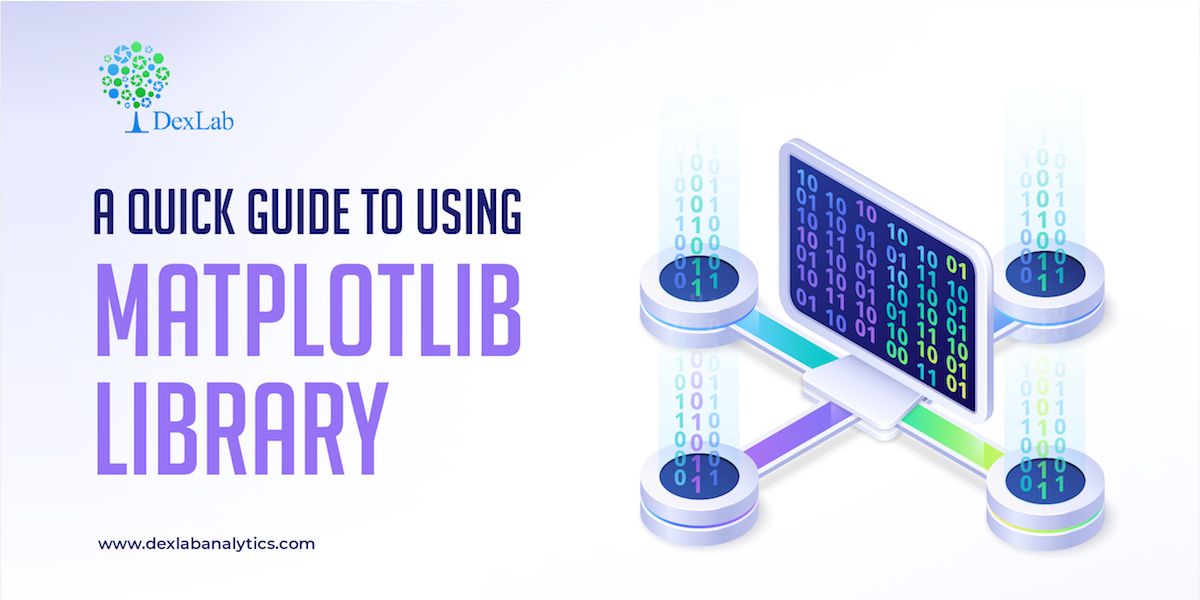Matplotlib is the “grandfather” library of data visualization with Python. It was created by John Hunter. He created it to try replicating MatLab’s (another programming language) plotting capabilities in Python. So, if you are already familiar with matlab, matplotlib will feel natural to you.

This library gives you the flexibility to plot the graphs the way you want. You can start with a blank canvas and plot the graph on that canvas wherever you want. You can make multiple plots on top of each other, change the line type, change the line color using predefined colors or hex codes, line width etc.#### Installation

Before you begin you’ll need to install matplotlib first by using the following code:-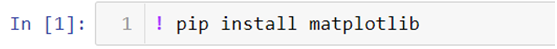There are two ways in which you can built matplotlib graphs:-

• Method based graphs
• Object-oriented graphs

#### Method Based Graphs in Matplotlib:-

There are pre-defined methods in matplotlib library which you can use to  create graphs directly using python language for example:-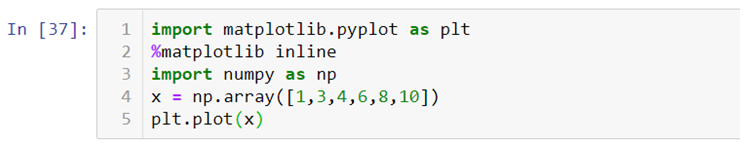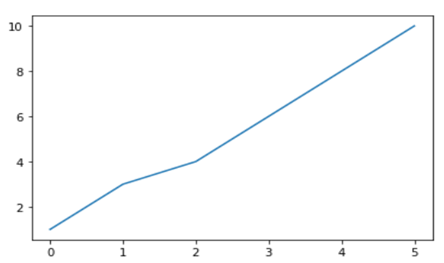Where, import matplotlib.pyplot as plt this code is used to import the library, %matplotlib inline is used to keep the plot within the parameters of the jupyter notebook, import numpy as np and x = np.array([1,3,4,6,8,10]) is used to import the numpy (numerical python) library and create an array x and plt.plot(x) is used to plot the distribution of the x variable.

We can also use .xlabel(), .ylabel() and .title() methods print x axis and y axis labels and title on the graph.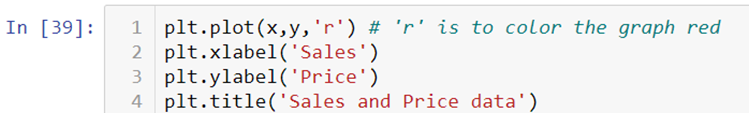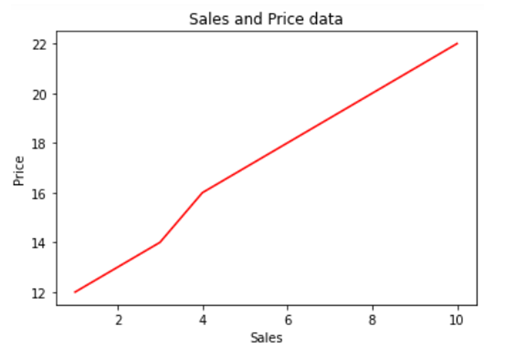If you want to add text within your graph you can either use .annotate() method or .text() method.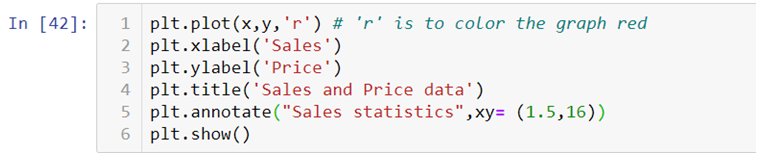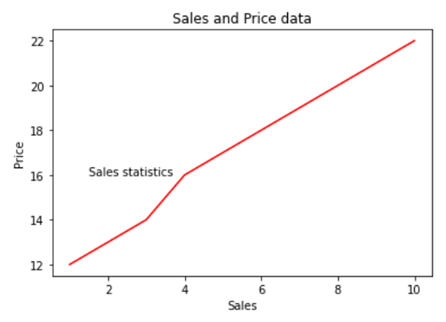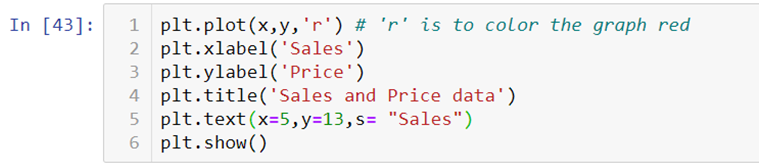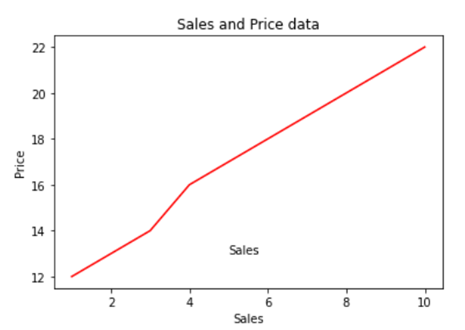#### Creating Multiplots

You can also create multiple plots by using .subplot() method by mentioning the number of rows and columns in which you want your graphs to be plotted. It works similar to the way you mention the number of rows and columns in a matrix.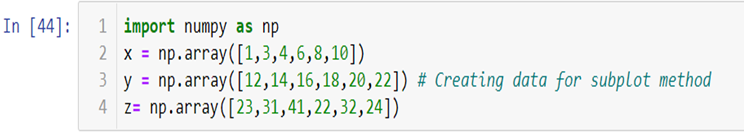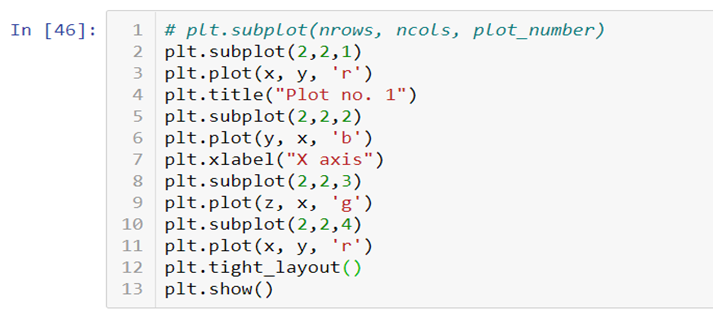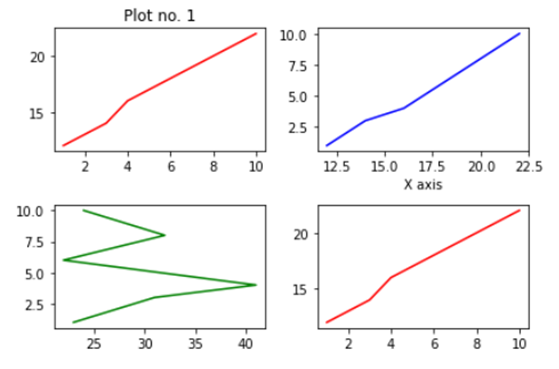You can add title, axis labels, texts etc., on each plot separately. In the end you can add .tight_layout() to solve the problem of overlapping of the graphs and to make the labels and scales visible.

Check out the video attached below to get an in-depth understanding of how Matplotlib works. This is a part of a visualization series using Python programming language. So, stay tuned for more updates. You can discover more such informative posts on the Dexlab Analytics blog.

.

+91 931 572 5902